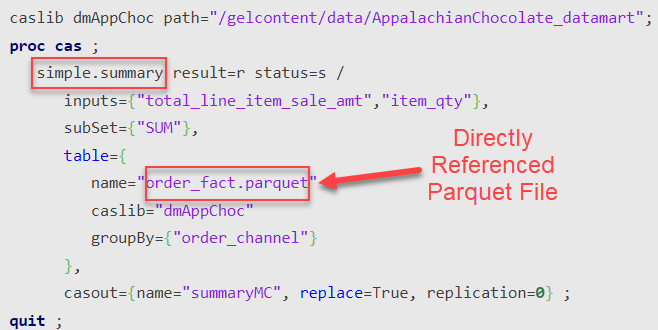## Processing Parquet: CAS vs Compute

Started ‎10-20-2022 by
Modified ‎12-07-2022 by
Views 1,462

As with most things these days, you have two options when it comes to processing parquet files, the CAS server and the traditional SAS engine now called "Compute." Let's see how we use both and any special considerations we should take into account in choosing one over the other.

### Performance Comparison -- Data Summarization

Examining basic data summarization, we see both engines performing exceptionally well and almost identically. Operating on the 5 million row, 26 column order_fact table parquet file both engines summarized 2 columns by 1 column in about 2 seconds. Performance drops modestly for both as variables are added to the analyses. Summarizing 4 columns by 4 columns takes about 5 seconds.

CAS' performance might surprise some here because of its need to load data prior to processing. However, as we can see below, the simple.Summary action references the parquet file directly. This triggers the parquet file to be loaded "transiently" into memory in its native parquet format and only the necessary fields are loaded. There is no expansion to the SASHDAT format.

#### CAS Code -- Simple.Summary#### Base SAS Code -- PROC MEANS

``````libname pqtdtm parquet "/gelcontent/data/AppalachianChocolate_datamart" ;
proc means data=pqtdtm.order_fact noprint;
var total_line_item_sale_amt item_qty;
class order_channel;
output out=summaryMC sum(total_line_item_sale_amt)= sum(item_qty)=;
run;``````

### Load Time and Size Comparison

Next we'll look at simply loading the parquet file to each server's native format, SAS7BDAT for Compute and DVR SASHDAT for CAS. We do this since we'll seldom process third party data in one step. Typically we augment it, enhance it, and transform it. This will typically require the data be transformed into the native format at some point. Additionally, certain CAS actions like DATA Step and FedSQL can not reference parquet files directly. So parquet files need to be loaded for their use.

CAS loads the 5 million row order_fact parquet file in ~15 seconds to DVR CAS table format which takes 0.44GB of space; Compute (base SAS) loads it in ~13 seconds to SAS7BDAT which takes 1GB of space.

### Conclusion

Both Compute and CAS read and process parquet files extremely efficiently. When it comes time to load the parquet data into CAS or SAS format for more complex processing however, CAS can store it more efficiently with DVR and can generally process it more efficiently as well.

### Code Used for this Analysis

``````*Create a large parquet table;

libname sasdtm "/gelcontent/data/AppalachianChocolate_datamart" ;
libname pqtdtm parquet "/gelcontent/data/AppalachianChocolate_datamart" ;

data order_fact;
set sasdtm.order_fact;
run;

data customer_dim;
set sasdtm.customer_dim;
run;

data pqtdtm.order_fact ;
set order_fact order_fact order_fact order_fact order_fact
order_fact order_fact order_fact order_fact order_fact ;
run ;

data pqtdtm.customer_dim;
set customer_dim;
run;

*Process the large parquet table with SAS;

*2 var, one class;
proc means data=pqtdtm.order_fact noprint;
var total_line_item_sale_amt item_qty;
class order_channel;
output out=summaryMC sum(total_line_item_sale_amt)= sum(item_qty)=;
run;

*4 var, 4 class;
proc means data=pqtdtm.order_fact noprint;
var total_line_item_sale_amt item_qty line_item_discount_amt list_price_amt;
class order_channel order_type retail_transaction_type_cd store_geo_id;
output out=summaryMC sum(total_line_item_sale_amt)= sum(item_qty)= sum(line_item_discount_amt)= sum(list_price_amt)=;
run;

proc datasets lib=work; run;

*Process the large parquet table with CAS;

cas mysession sessopts=(metrics=true);
caslib _all_ assign;
caslib dmAppChoc path="/gelcontent/data/AppalachianChocolate_datamart";

*2 var, 1 class;
proc cas ;
simple.summary result=r status=s /
inputs={"total_line_item_sale_amt","item_qty"},
subSet={"SUM"},
table={
name="order_fact.parquet"
caslib="dmAppChoc"
groupBy={"order_channel"}
},
casout={name="summaryMC", replace=True, replication=0} ;
quit ;

* 4 var, 4 class;
proc cas ;
simple.summary result=r status=s /
inputs={"total_line_item_sale_amt","item_qty", "line_item_discount_amt","list_price_amt"},
subSet={"SUM"},
table={
name="order_fact.parquet"
caslib="dmAppChoc"
groupBy={"order_channel" "order_type" "retail_transaction_type_cd" "store_geo_id"}
},
casout={name="summaryMC", replace=True, replication=0} ;
quit ;

proc cas;
table.loadtable caslib="dmAppChoc" path="order_fact.parquet" casout={caslib="dmAppChoc" name="order_fact" replication=0};
quit;

proc cas;
table.tabledetails caslib="dmAppChoc" name="order_fact" level="sum";
quit;

data sasdm.order_fact;
set pqtdtm.order_fact;
run;

proc datasets lib=sasdm; run;
proc contents data=sasdm.order_fact; run;

cas mysession terminate;``````

Find more articles from SAS Global Enablement and Learning here.

Version history
Last update:
‎12-07-2022 06:55 AM
Updated by:Contributors
Article Labels
Article Tags